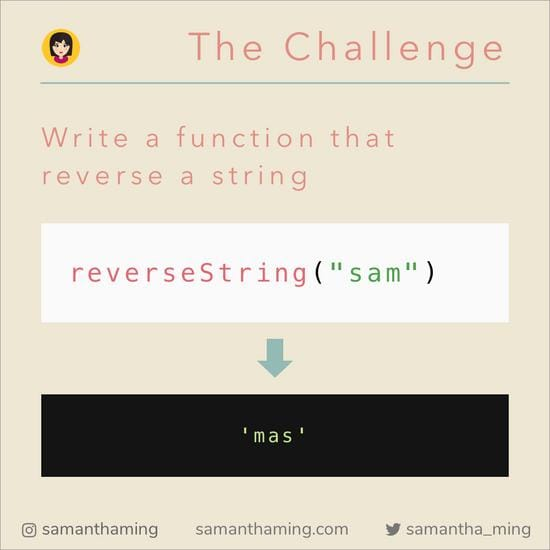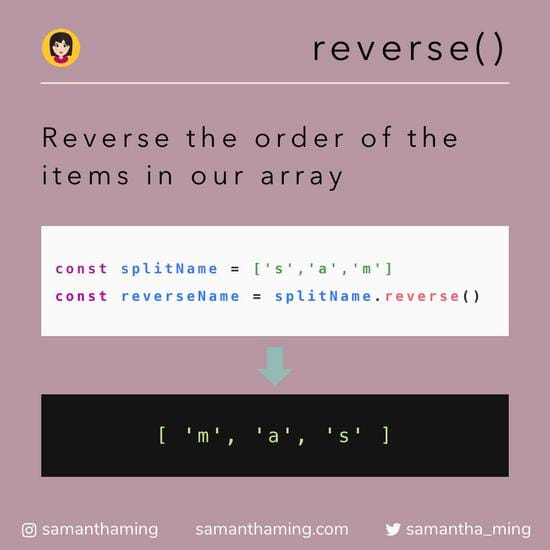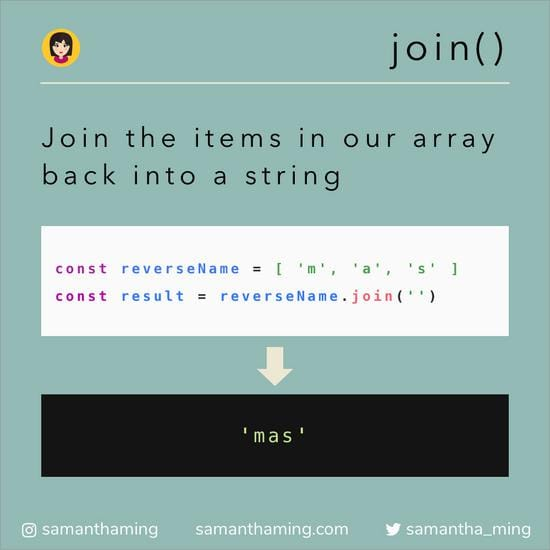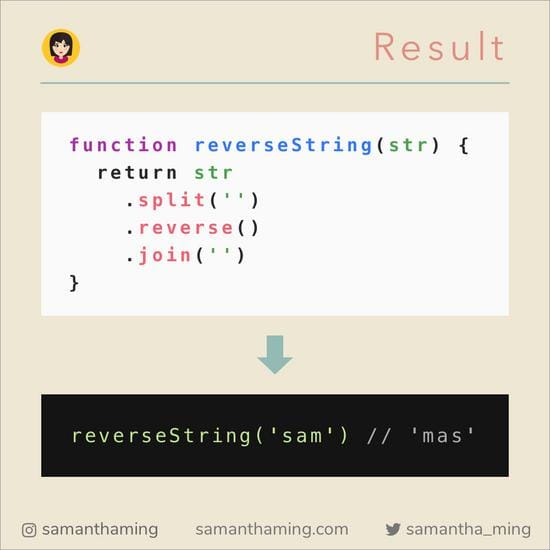# # How to Reverse a String in JavaScript

Welcome to the first Mini Code Tutorial! @fototeka.inikas sent a DM to me saying I should start a shorter tutorial series. He said I would be number 1 if I did it 🤣. So let’s see if he’s right 😂. JK. Doing this shorter tutorial have been on my list for awhile. But have you every had a huge list of things to do, and you never end up doing any of it all. Anyways, I’m happy for the nudge!

In today's lesson, we're going to learn how to reverse a string! Remember in my Web Basics Series, we covered these 3 methods. Let's now apply them and solve this popular algorithm challenge 👍## # The Challenge

Write a function that reverse a string.## # 1. `split()`

In JavaScript, there is no built-in method to reverse a string. There is however, a built-in method to reverse an array. So the first step is convert our string into an array.

Split our string into an array of letters.## # 2. `reverse()`

Excellent, now that we have an array of letters. We can call our built-in array method to reverse the order.

Reverse the order of the items in our array.## # 3. `join()`

Now that our array contains the reversed letters. Let's convert the array back into a string.

Join the items in our array back into a string.## # Final Solution

And there we have it! We can chain our methods together to a nice function. Congratulation, you have now learned how to reverse a string in JavaScript 🥳## # More Solutions

Using `reverse`

``````function reverseString(str) {
return str
.split('')
.reverse()
.join('');
}
``````

Using `reduce`

``````function reverseString(str) {
return [...str].reduce((accumulator, current) => {
return current + accumulator;
});

// OR One-Liner
// return [...str].reduce((accumulator, current) => current + accumulator)
}
``````

Using `reduceRight`

``````function reverseString(str) {
return [...str].reduceRight((accumulator, current) => accumulator + current);
}
``````

Using `for loop`

``````function reverseString(str) {
let result = '';

for (let i = str.length - 1; i >= 0; i--) {
result += str[i];
}

return result;
}
``````

Using `sort`

``````function reverseString(str) {
return str
.split('')
.sort(() => 1)
.join('');
}
``````

Using `recursion`

``````function reverseString(str = '') {
const [head = '', ...tail] = str;

if (tail.length) {